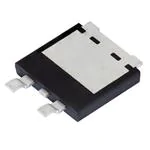< id="6e2kg">< id="6e2kg">< id="6e2kg">< id="6e2kg">< id="6e2kg">< id="6e2kg">< id="6e2kg">< id="6e2kg">< id="6e2kg">< id="6e2kg">< id="6e2kg">< id="6e2kg">< id="6e2kg">< id="6e2kg">< id="6e2kg">< id="6e2kg">< id="6e2kg">< id="6e2kg">< id="6e2kg">< id="6e2kg">< id="6e2kg">< id="6e2kg">< id="6e2kg">< id="6e2kg">< id="6e2kg">< id="6e2kg">< id="6e2kg">< id="6e2kg">< id="6e2kg">< id="6e2kg">< id="6e2kg">< id="6e2kg">< id="6e2kg">< id="6e2kg">< id="6e2kg">< id="6e2kg">< id="6e2kg">< id="6e2kg">< id="6e2kg">< id="6e2kg">< id="6e2kg">< id="6e2kg">< id="6e2kg">< id="6e2kg">< id="6e2kg">< id="6e2kg">< id="6e2kg">< id="6e2kg">< id="6e2kg">< id="6e2kg"> < id="6e2kg">< id="6e2kg">< id="6e2kg">< id="6e2kg">< id="6e2kg">< id="6e2kg">< id="6e2kg">< id="6e2kg">< id="6e2kg">< id="6e2kg">< id="6e2kg">< id="6e2kg">< id="6e2kg">< id="6e2kg">< id="6e2kg">< id="6e2kg">< id="6e2kg">< id="6e2kg">< id="6e2kg">< id="6e2kg">< id="6e2kg">< id="6e2kg">< id="6e2kg">< id="6e2kg">< id="6e2kg">< id="6e2kg">< id="6e2kg">< id="6e2kg">< id="6e2kg">< id="6e2kg">< id="6e2kg">< id="6e2kg">< id="6e2kg">< id="6e2kg">< id="6e2kg">< id="6e2kg">< id="6e2kg">< id="6e2kg">< id="6e2kg">< id="6e2kg">< id="6e2kg">< id="6e2kg">< id="6e2kg">< id="6e2kg">< id="6e2kg">< id="6e2kg">< id="6e2kg">< id="6e2kg">< id="6e2kg">< id="6e2kg"> < id="6e2kg">< id="6e2kg">< id="6e2kg">< id="6e2kg">< id="6e2kg">< id="6e2kg">< id="6e2kg">< id="6e2kg">< id="6e2kg">< id="6e2kg">< id="6e2kg">< id="6e2kg">< id="6e2kg">< id="6e2kg">< id="6e2kg">< id="6e2kg">< id="6e2kg">< id="6e2kg">< id="6e2kg">< id="6e2kg">< id="6e2kg">< id="6e2kg">< id="6e2kg">< id="6e2kg">< id="6e2kg">< id="6e2kg">< id="6e2kg">< id="6e2kg">< id="6e2kg">< id="6e2kg">< id="6e2kg">< id="6e2kg">< id="6e2kg">< id="6e2kg">< id="6e2kg">< id="6e2kg">< id="6e2kg">< id="6e2kg">< id="6e2kg">< id="6e2kg">< id="6e2kg">< id="6e2kg">< id="6e2kg">< id="6e2kg">< id="6e2kg">< id="6e2kg">< id="6e2kg">< id="6e2kg">< id="6e2kg">< id="6e2kg">型号： V40DM100C-M3/I 厂商： Vishay Semiconductors 标准：分类： 半导体 ， 二极管，整流器 - 阵列 描述： 肖特基二极管与整流器 100v 40a smpd(TO-263ac) 报错 收藏 赞

Datasheet下载地址

V40DM100C-M3/I的详细信息

制造商： Vishay 肖特基二极管与整流器 详细信息 Schottky Rectifiers SMD/SMT TO-263AC-3 40 A 100 V 730 mV 240 A Dual 700 uA - 40 C + 175 C V40DM Cut Tape MouseReel Reel Vishay Semiconductors Schottky Diodes & Rectifiers 2000 Diodes & Rectifiers TMBS eSMP